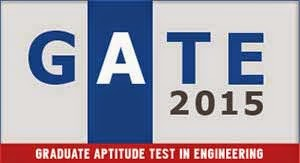GATE 2015 SYLLABUS FOR Civil Engineering (CE)

### Engineering Mathematics

Linear Algebra:  Matrix algebra, Systems of linear equations, Eigen values and eigenvectors.Calculus:  Functions  of  single  variable,  Limit,  continuity  and  differentiability,  Mean  value  theorems,   Evaluation   of   definite   and   improper   integrals,   Partial   derivatives,   Total  derivative,   Maxima   and   minima,   Gradient,   Divergence   and   Curl,   Vector   identities,  Directional  derivatives,  Line,  Surface  and  Volume  integrals,  Stokes,  Gauss  and  Green’s  theorems.

Differential  equations:   First  order  equations  (linear  and  nonlinear),  Higher  order  linear  differential equations with constant coefficients, Cauchy’s and Euler’s equations, Initial and  boundary value problems, Laplace transforms, Solutions of one dimensional heat and wave  equations and Laplace equation.  Complex  variables:   Analytic  functions,  Cauchy’s  integral  theorem,  Taylor  and  Laurent  series.

Probability and Statistics:  Definitions of probability and sampling theorems, Conditional  probability,  Mean,  median,  mode  and  standard  deviation,  Random  variables,  Poisson,  Normal and Binomial distributions.  Numerical  Methods:   Numerical  solutions  of  linear  and  non-linear  algebraic  equations  Integration   by   trapezoidal   and   Simpson’s   rule,   single   and   multi-step   methods   for  differential equations.

### Structural Engineering

Mechanics : Bending moment and shear force in statically determinate beams. Simple stress  and  strain  relationship:  Stress  and  strain  in  two  dimensions,  principal  stresses,  stress  transformation,   Mohr’s   circle.   Simple   bending   theory,   flexural   and   shear   stresses,  unsymmetrical  bending,  shear  centre.  Thin  walled  pressure  vessels,  uniform  torsion,  buckling of column, combined and direct bending stresses.

Structural  Analysis :  Analysis  of  statically  determinate  trusses,  arches,  beams,  cables  and  frames,   displacements   in   statically   determinate   structures   and   analysis   of   statically  indeterminate  structures  by  force/  energy  methods,  analysis  by  displacement  methods  (slope  deflection  and  moment  distribution  methods),  influence  lines  for  determinate  and  indeterminate structures. Basic concepts of matrix methods of structural analysis.

Concrete  Structures :  Concrete  Technology-  properties  of  concrete,  basics  of  mix  design.  Concrete design- basic working stress and limit state design concepts, analysis of ultimate  load capacity and design of members subjected to flexure, shear, compression and torsion  by limit state methods. Basic elements of prestressed concrete, analysis of beam sections at  transfer and service loads.

Steel  Structures :  Analysis  and  design  of  tension  and  compression  members,  beams  and  beam-    columns,    column    bases.    Connections-    simple    and    eccentric,    beam–column  connections, plate girders and trusses.Plastic analysis of beams and frames.

### Geotechnical Engineering

Soil   Mechanics :   Origin   of   soils,   soil   classification,   three-phase   system,   fundamental  definitions,  relationship  and  interrelationships,  permeability  &seepage,  effective  stress  principle, consolidation, compaction, shear strength.

Foundation Engineering : Sub-surface investigations- scope, drilling bore holes, sampling,  penetration tests, plate load test. Earth pressure theories, effect of water table, layered soils.  Stability   of   slopes-infinite   slopes,   finite   slopes.   Foundation   types-foundation   design  requirements. Shallow foundations-bearing capacity, effect of shape, water table and other  factors, stress distribution, settlement analysisinsands& clays. Deep foundations–pile types,  dynamic &static formulae, load capacity of piles in sands &clays, negative skin friction.

### Water Resources Engineering

Fluid  Mechanics  and  Hydraulics :  Properties  of  fluids,  principle  of  conservation  of  mass,  momentum,    energy    and    corresponding    equations,    potential    flow,    applications    of  momentum  and  Bernoulli’s  equation,  laminar  and  turbulent  flow,  flow  in  pipes,  pipe  networks.  Concept  of  boundary  layer  and  its  growth.Uniform  flow,  critical  flow  and  gradually  varied  flow  in  channels,  specific  energy  concept,  hydraulic  jump.Forces  on  immersed  bodies,  flow  measurements  in  channels,  tanks  and  pipes.Dimensional  analysis  and hydraulic modeling. Kinematics of flow, velocity triangles and specific speed of pumps  and turbines.

Hydrology:     Hydrologic    cycle,    rainfall,    evaporation,    infiltration,    stage    discharge  relationships, unit hydrographs, flood estimation, reservoir capacity, reservoir and channel  routing. Well hydraulics.

Irrigation:  Duty, delta, estimation of evapo-transpiration. Crop water requirements. Design  of: lined and unlined canals, waterways, head works, gravity dams and spillways. Design of  weirs   on   permeable   foundation.Types   of   irrigation   system,   irrigation   methods.Water  logging and drainage, sodic soils.  Environmental Engineering

Water  requirements :  Quality  standards,  basic  unit  processes  and  operations  for  water  treatment.  Drinking  water  standards,  water  requirements,  basic  unit  operations  and  unit  processes   for   surface   water   treatment,   distribution   of   water.   Sewage   and   sewerage  treatment,  quantity  and  characteristics  of  wastewater.  Primary,  secondary  and  tertiary  treatment   of   wastewater,   sludge   disposal,   effluent   discharge   standards.   Domestic  wastewater  treatment,  quantity  of  characteristics  of  domestic  wastewater,  primary  and  secondary  treatment  Unit  operations  and  unit  processes  of  domestic  wastewater,  sludge  disposal.

Air Pollution:  Types of pollutants, their sources and impacts, air pollution meteorology, air  pollution control, air quality standards and limits.

Municipal Solid Wastes : Characteristics, generation, collection and transportation of solid  wastes, engineered systems for solid waste management (reuse/ recycle, energy recovery,  treatment and disposal).

Noise  Pollution :  Impacts  of  noise,  permissible  limits  of  noise  pollution,  measurement  of  noise and control of noise pollution.

### Transportation Engineering

Highway  Planning:  Geometric  design  of  highways,  testing  and  specifications  of  paving  materials, design of flexible and rigid pavements.

Traffic  Engineering :  Traffic  characteristics,  theory  of  traffic  flow,  intersection  design,  traffic signs and signal design, highway capacity.

### Surveying

Importance  of  surveying,  principles  and  classifications,  mapping  concepts,  coordinate  system,  map  projections,  measurements  of  distance  and  directions,  leveling,  theodolite  traversing, plane table surveying, errors and adjustments, curves.